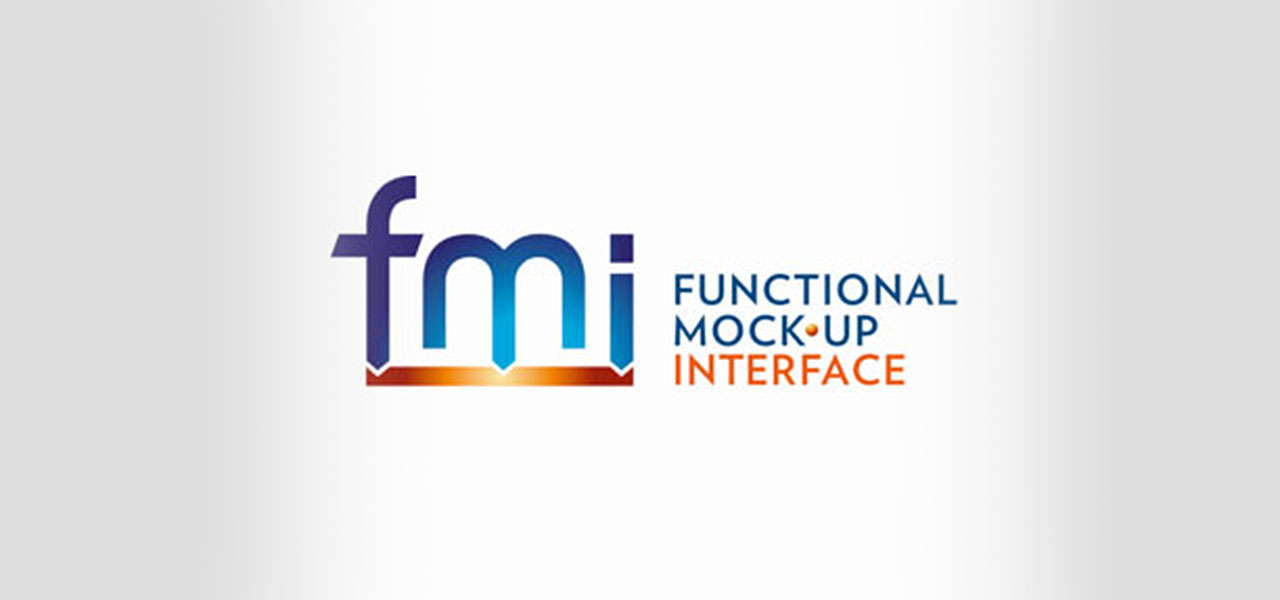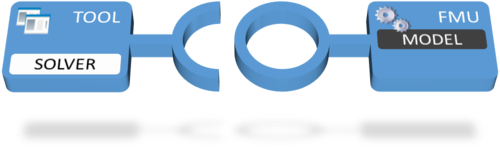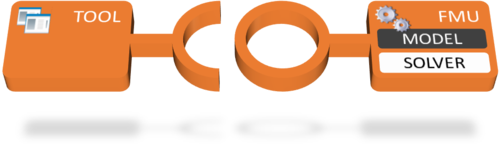The Functional Mock-up Interface (FMI) standard supports two flavors of Functional Mock-up Units (FMU), including: Co-Simulation (CS) and Model Exchange (ME). But, do you know the difference?

As an expert in the standard it’s my goal to help users understand the commonalities and differences – getting you to a point in which you’re choosing the best possible technique to achieve your goal! Let’s first look at what the two kinds of FMUs have in common.

If the FMI standard is brand new to you, you may want to read this first: What is FMI?

## What are the similarities?

A common source of confusion lies in the names of the two kinds of FMU: Model Exchange and Co-Simulation. Because in fact, both ME and CS FMUs can be used both for exchanging models and for simulating multiple models together. More specifically:

1. Both CS and ME FMUs are files with the extension .fmu.
2. Both are  used to model dynamical systems that can have inputs, outputs, parameters, and other variables.
3. Both types can be simulated, meaning that you compute how the state of the modeled system evolves over time.
4. Both CS and ME FMUs can be used for model exchange, that is, exporting a model from one tool and importing it into another.
5. And both CS and ME FMUs can be used for co-simulation, in the sense of simulating multiple models together.

So you may be asking…..then what is the difference?

## What are the differences?

The difference between the two kinds consist of how the importing tool makes the FMU step forward in time.

Models that represent physical quantities such as temperature or velocity are generally described by differential equations. The way that the quantities change with time depend on the value of the quantities themselves (the state), and what external influence they are subjected to (the inputs).

Let’s take the following example: A very simple mathematical model for how the temperature of a house evolves can be written as:

dThouse/dt = k1*(Tout-Thouse) k2*BoilerPower k3

Here, the rate of change of the quantity (dThouse/dt) depends on the state (Thouse) and the inputs (Tout and BoilerPower). k1, k2, and k3 are parameters that do not change with time. To compute how the temperature in the house changes with time, the differential equation needs to be hooked up to a numerical solver.

When you simulate a CS FMU, the numerical solver is embedded and supplied by the exporting tool. The importing tool will set the inputs, tell the FMU to step forward a given time, and then read the outputs.

When you simulate an ME FMU, the numerical solver is supplied by the importing tool. The FMU provides functions to set the state and inputs, and to compute the state derivatives (i.e., the left hand side of the equation). The solver in the importing tool will determine what time steps to use, and how to compute the state at the next time step.Model ExchangeCo-Simulation

Based on the FMI 2.0 standard, an FMU may be both an CS and ME FMU. That means that it provides both the ME functions that can be hooked up to a solver in the importing tool, and an internal solver if you choose to use the FMU as CS.

## Functional Mock-up Unit: Which should I use?

This is a question that I get asked all the time. And the answer is: it depends. To understand the pros and cons of the two types, I like to compare to vector and bitmap graphics.

• CS is more like bitmap graphics (like .jpg or .png). The rendering is mainly determined by the exporting tool. You can extract data at a grid of points but not continuously. The solver settings can sometimes be changed through parameters in the FMU, but you cannot change to a different solver.
• ME is the equivalent of vector graphics (like .svg or .eps); it provides functions that directly access the underlying mathematical model and can be manipulated by the importing tool in a way that is adapted to the context where it is to be used. Zooming in or interpolating? No problem.

Does that mean that you should always use ME? No. Just like you don’t always use vector graphics formats. Some reasons to choose CS include:

• Not all tools support both types. Support for CS is more common than for ME.
• The numerics of the model may need a specific solver that is available in the exporting tool but not in the importing one.
• The FMU may represent a sampled data system (such as signal processing or control algorithms), that is not governed by differential equations and thus more naturally represented as a CS FMU.
• The exporting tool may have a more efficient implementation of the solver in terms of memory usage, execution time, etc.

A general guideline could be to try and see if ME works. If you work with “simple” models (small, linear, non-stiff), ME is often an excellent choice because it allows more flexibility. If you have models that take in the order of minutes or more to simulate (even in native tools with specialized solvers) ME could likely be challenging and you should consider CS instead.

Check with tool vendors for both importing and exporting tools in your intended tool chain to see what options are available. An overview for different tools is available here.